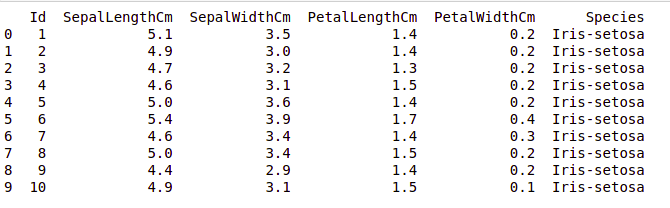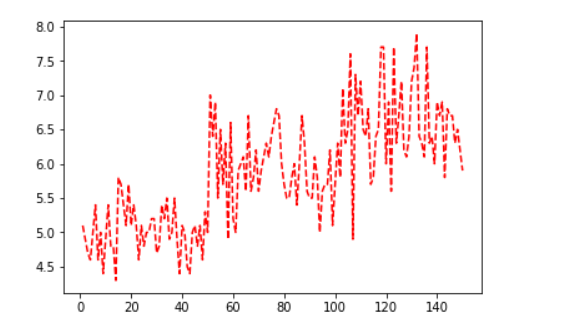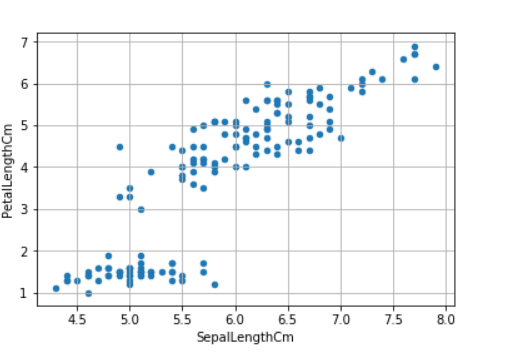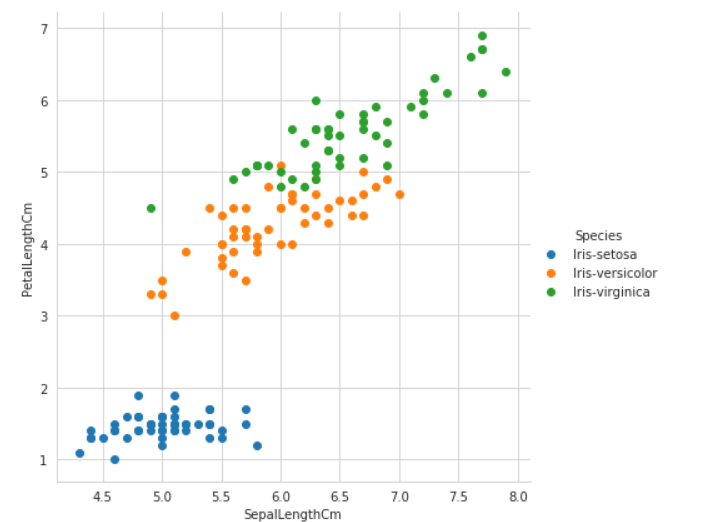# Plotting graph For IRIS Dataset Using Seaborn And Matplotlib

Matplotlib.pyplot library is most commonly used in Python in the field of machine learning. It helps in plotting the graph of large dataset. Not only this also helps in classifying different dataset. It can plot graph both in 2d and 3d format. It has a feature of legend, label, grid, graph shape, grid and many more that make it easier to understand and classify the dataset.

Seaborn provides a beautiful with different styled graph plotting that make our dataset more distinguishable and attractive.

### Installation

To install the package write the below code in terminal of ubuntu/Linux or Window Command prompt.

```pip install matplotlib
pip install seaborn```

```Attribute Information:
-> sepal length in cm
-> sepal width in cm
-> petal length in cm
-> petal width in cm
-> class:
Iris Setosa
Iris Versicolour
Iris Virginica

Number of Instances: 150

Summary Statistics:
Min  Max   Mean    SD   Class Correlation
sepal length: 4.3  7.9   5.84  0.83    0.7826
sepal width: 2.0  4.4   3.05  0.43   -0.4194
petal length: 1.0  6.9   3.76  1.76    0.9490  (high!)
petal width: 0.1  2.5   1.20  0.76    0.9565  (high!)

Class Distribution: 33.3% for each of 3 classes.```

## Python3

 `import` `numpy as np ` `import` `pandas as pd ` `import` `matplotlib.pyplot as plt `   `data ``=` `pd.read_csv(``"Iris.csv"``) ` `  `  `print` `(data.head(``10``))`

Output:Plotting Using Matplotlib

## Python3

 `import` `pandas as pd` `import` `matplotlib.pyplot as plt` `iris ``=` `pd.read_csv(``"Iris.csv"``)`   `plt.plot(iris.``Id``, iris[``"SepalLengthCm"``], ``"r--"``)` `plt.show`Scatter Plot

## Python3

 `iris.plot(kind ``=``"scatter"``,` `          ``x ``=``'SepalLengthCm'``,` `          ``y ``=``'PetalLengthCm'``)` `plt.grid()`Plotting using Seaborn

## Python3

 `import` `seaborn as sns`   `iris ``=` `sns.load_dataset(``'iris'``)`   `# style used as a theme of graph ` `# for example if we want black ` `# graph with grid then write "darkgrid"` `sns.set_style(``"whitegrid"``)`   `# sepal_length, petal_length are iris` `# feature data height used to define` `# Height of graph whereas hue store the` `# class of iris dataset.` `sns.FacetGrid(iris, hue ``=``"species"``, ` `              ``height ``=` `6``).``map``(plt.scatter, ` `                              ``'sepal_length'``, ` `                              ``'petal_length'``).add_legend()`Whether you're preparing for your first job interview or aiming to upskill in this ever-evolving tech landscape, GeeksforGeeks Courses are your key to success. We provide top-quality content at affordable prices, all geared towards accelerating your growth in a time-bound manner. Join the millions we've already empowered, and we're here to do the same for you. Don't miss out - check it out now!

Previous
Next